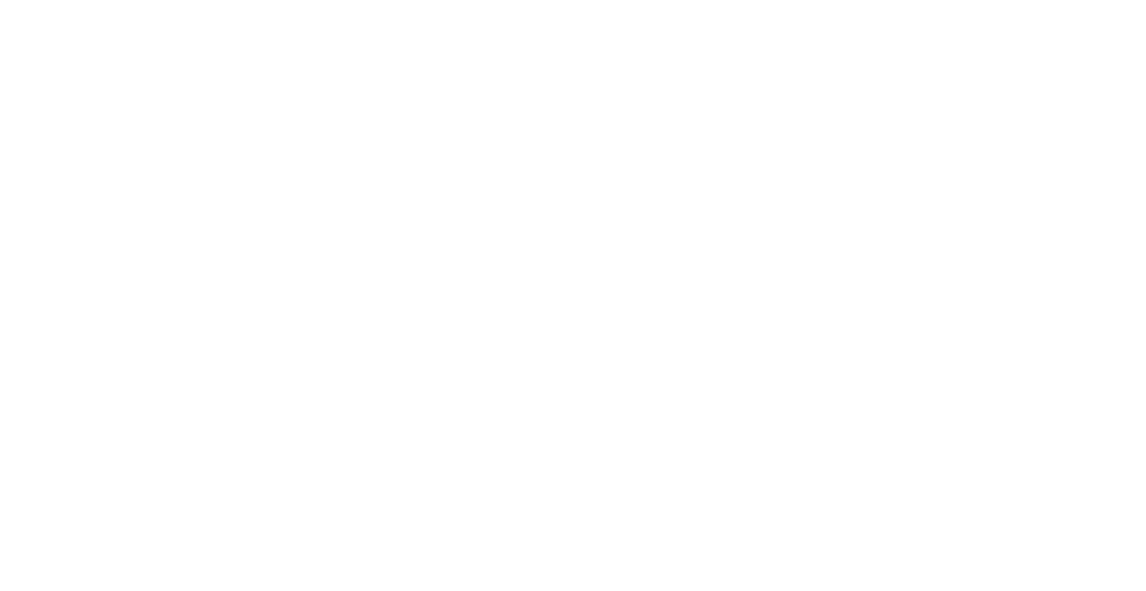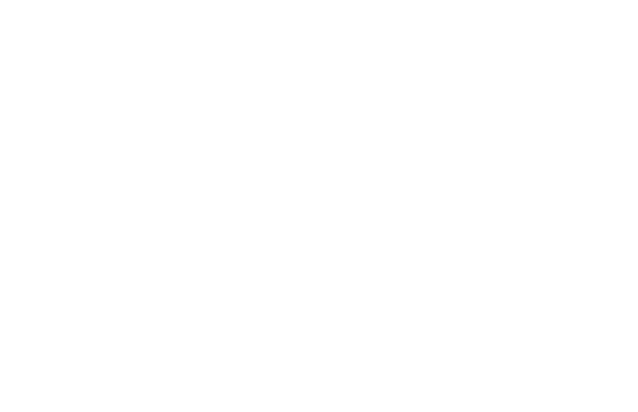##Painting is one of the most essential steps to be done after the construction of any building/house. Painting is done to protect the surface of the walls as well as to increase the appearance.

In this article, I will discuss how to calculate the quantity of paint required in a building.

Before starting the calculation let me tell you one important thing the quantity of paint is calculated in gallons.

There are two types of gallons

US gallon and Uk gallon.

1 US gallon = 3.785 litres

1 Uk gallon = 4.546 litres

In this calculation, we will use US gallons.Let the length of the wall = 15 feet

The height of the wall = 12 feet.

Area of the wall 15 x 12 = 180 sft.

Thumb Rule For Paint Calculation

Generally,

• 1 gallon of paint can cover up to 350 Sft area of Wet-wall
• 1 gallon of paint can cover up to 200 Sft area of Dry-wall

Paint Quantity Of Wet wall

Area of wet wall =180 Sft

Paint covered area = 350 Sft

Quantity of paint = 180/350 = 0.514 gallon

Let the price of 1-gallon paint = 20\$ (it is different from a country to another country (15-40))

Price of 0.514 gallon paint = 0.514 x 20 =  10.28\$

Paint Quantity Of Dry Wall

Area of dry wall = 180 Sft.

Pain covered area = 200

Quantity of paint = 180/200 =0.9 gallon.

Price of 0.9 gallon paint = 0.9 x 20 = 18 \$

Note:

1. If the wall has any openings, subtract the area of openings from the total area & then calculate paint quantity.

Click Here To See Easiest Method To Calculate Number Of Bricks In A Wall